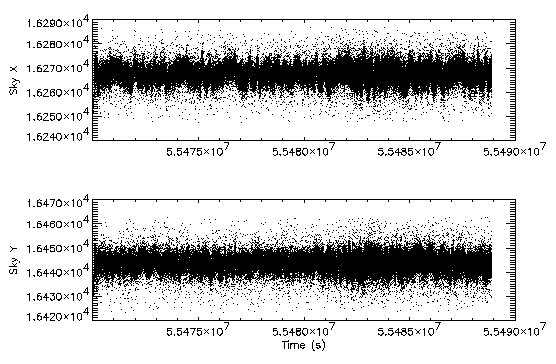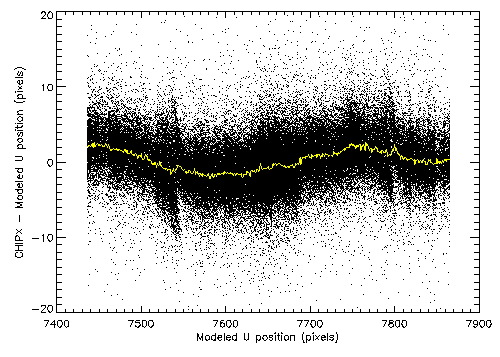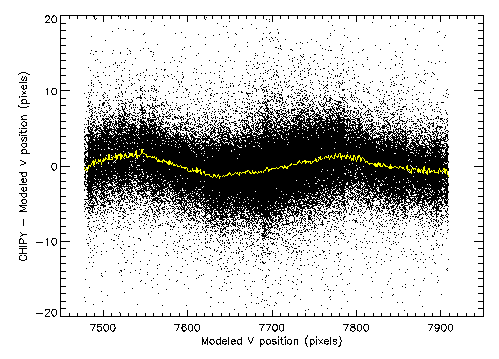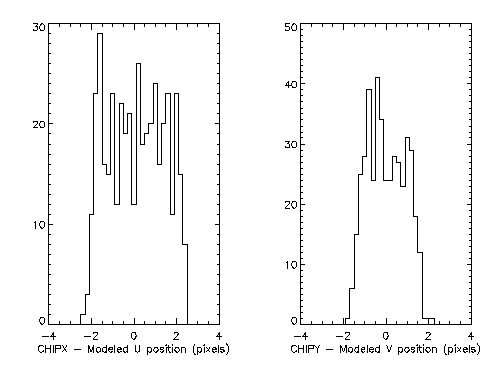# Blur from Residual Errors in HRC Event Position Reconstruction

## Introduction

The HRC event positions are not telemetered to the ground but must be reconstructed from data generated by the HRC crossed-grid charge detector (CGCD) (see memo HRC Position Logic). In reconstructing the event positions corrections for ringing in amplifiers and degapping are applied. The detector positions that result are then combined with information on the position and motion of the detector behind the HRMA and with the telescope aspect solution to determine a celestial location for each event. The quality of the resulting images is determined by the combination of "blur" from several sources: the PSF of the HRMA, the aspect solution, the intrinsic resolution of the HRC detector, and the HRC event position reconstruction. In this memo I will address the last of these contributors.

Figure 1 demonstrates the result of event position reconstruction errors. Using the most recent processing of data from ObsID 1385 (a ~19ks observation of AR Lac), I have selected events from hrcf01385_000N004_evt1.fits within a 20 pixel radius centered on the source and plotted the "Sky" X and Y positions versus time. The wobble that is apparent in the mean position as a function of time is indicative of an error in either the aspect reconstruction or the HRC event position reconstruction. Since this sort of wobble is not observed in ACIS observations, it is logical to attribute it to dithering over the residual errors in the determination of HRC event positions.Figure 1: Events within 20 pixels of AR Lac: X and Y plotted versus time.

## HRC Position Errors

Residual errors in the HRC positions are the likely cause of the apparent wobble observed in Figure 1. We can use the spacecraft dither as a probe of the position errors as a function of detector position by using the aspect solution to model the expected location of the source on the HRC detector. Starting from the aspect solution file, pcadf055469783N003_asol1.fits, and the nominal pointing direction, I modeled the expected time history of the location of AR Lac on the HRC detector (U and V axes) including the motion of the detector behind the HRMA. Then for each event I calculated the model position by linear interpolation of location time history. Plotted in figure 2 is the deviation of the corrected (de-rung and degapped) event position from the modeled event position as a function of modeled position for the HRC U-axis; figure 3 is similar but for the V-axis. There is a natural width to the deviations which is due to the HRMA, aspect reconstruction, and intrinsic HRC detector blur but the systematic wave as a function of modeled position is indicative of errors in the HRC event position reconstruction. The size of this systematic error is shown in the over-plotted yellow curve which is the mean of the observed deviation as a function of modeled position.Figure 2: Observed deviations from modeled event position on the HRC U-axis. The yellow curve is the average deviation at each modeled position.Figure 3: Observed deviations from modeled event position on the HRC V-axis. The yellow curve is the average deviation at each modeled position.

Figure 4 shows a plot of the histograms of the mean deviations. The additional blur caused by residual errors HRC event position reconstruction could be as large as 3-4 pixels or 0.4-0.5 arcsec. The actual additional blur that is observed will depend on how these distributions are sampled.Figure 4: Histograms of the deviations from modeled event positions for the U-axis (left) and the V-axis (right).

## Conclusions

The fact that there is a systematic trend in the deviations from modeled position as a function of modeled positions demonstrates that HRC event position reconstruction is a significant contributor to the overall PSF. It may be possible to reduce its contribution through improvements to the ringing or degapping corrections but this will require further study.

email Mike Juda